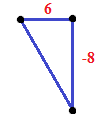### Home > AC > Chapter 8 > Lesson 8.2.4 > Problem8-81

8-81.

Find the equation of the line through the points $\left(6,-8\right)$ and $\left(0,0\right)$.

1. What is the slope of the line?

2. Is the point $\left(3,-4\right)$ on the line? How can you tell?

Graph the points and make a slope triangle to find the slope.Substitute the coordinates into the equation to see if the point is on the line.